OXIDATION OF ALDEHYDES AND KETONES

```
```

This page looks at ways of distinguishing between aldehydes and ketones using oxidising agents such as acidified potassium dichromate(VI) solution, Tollens' reagent, Fehling's solution and Benedict's solution.

```
```

Background

Why do aldehydes and ketones behave differently?

You will remember that the difference between an aldehyde and a ketone is the presence of a hydrogen atom attached to the carbon-oxygen double bond in the aldehyde. Ketones don't have that hydrogen.

```
``````
```

The presence of that hydrogen atom makes aldehydes very easy to oxidise. Or, put another way, they are strong reducing agents.

Note:  If you aren't sure about oxidation and reduction, it would be a good idea to follow this link to another part of the site before you go on. Alternatively, come back to this link if you feel you need help later on in this page.

Because ketones don't have that particular hydrogen atom, they are resistant to oxidation. Only very strong oxidising agents like potassium manganate(VII) solution (potassium permanganate solution) oxidise ketones - and they do it in a destructive way, breaking carbon-carbon bonds.

Provided you avoid using these powerful oxidising agents, you can easily tell the difference between an aldehyde and a ketone. Aldehydes are easily oxidised by all sorts of different oxidising agents: ketones aren't.

You will find details of these reactions further down the page.

```
```

What is formed when aldehydes are oxidised?

It depends on whether the reaction is done under acidic or alkaline conditions. Under acidic conditions, the aldehyde is oxidised to a carboxylic acid. Under alkaline conditions, this couldn't form because it would react with the alkali. A salt is formed instead.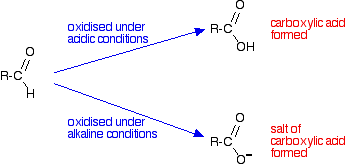Note:  In the case of methanal, HCHO, the oxidation goes further. The methanoic acid or methanoate ions formed are easily oxidised to carbon dioxide and water.

```
```

Building equations for the oxidation reactions

If you need to work out the equations for these reactions, the only reliable way of building them is to use electron-half-equations.

The half-equation for the oxidation of the aldehyde obviously varies depending on whether you are doing the reaction under acidic or alkaline conditions.

Under acidic conditions it is:. . . and under alkaline conditions:Note:  These electron-half-equations are quite easy to work out from scratch. There isn't any real need to remember them. Follow this link if you aren't sure how to do it, or if you aren't confident about using these equations. These particular examples aren't covered, but the technique is exactly the same whatever the equation.

Because I am going to be using electron-half-equations quite a lot on the rest of this page, it would definitely be worth following this link if you aren't happy about them.

These half-equations are then combined with the half-equations from whatever oxidising agent you are using. Examples are given in detail below.

```
```

Specific examples

In each of the following examples, we are assuming that you know that you have either an aldehyde or a ketone. There are lots of other things which could also give positive results.

Note:  Follow this link to find out how to test for the carbon-oxygen double bond in aldehydes and ketones.

Assuming that you know it has to be one or the other, in each case, a ketone does nothing. Only an aldehyde gives a positive result.

```
```

Using acidified potassium dichromate(VI) solution

A small amount of potassium dichromate(VI) solution is acidified with dilute sulphuric acid and a few drops of the aldehyde or ketone are added. If nothing happens in the cold, the mixture is warmed gently for a couple of minutes - for example, in a beaker of hot water.

 ketone No change in the orange solution. aldehyde Orange solution turns green.
```
```

The orange dichromate(VI) ions have been reduced to green chromium(III) ions by the aldehyde. In turn the aldehyde is oxidised to the corresponding carboxylic acid.

The electron-half-equation for the reduction of dichromate(VI) ions is:Combining that with the half-equation for the oxidation of an aldehyde under acidic conditions:. . . gives the overall equation: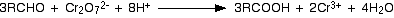Note:  You may wonder why I have gone to all the trouble of working out a complete equation for this reaction (and the next ones) rather than using symbols like [O] which are frequently used in organic chemistry.

The problem is that what is important in using these reactions as tests is the colour change in the oxidising agent. In this particular reaction, you have to explain, for example, why the solution turns green. Any equation that you write has got to show the production of the chromium(III) ions.

If you aren't sure exactly how the two half-equations are combined to give the final equation, follow the last link (further up the page) to learn how to do it - and then come back and have a go yourself to check that you can get the right answer.

```
```

Using Tollens' reagent (the silver mirror test)

Tollens' reagent contains the diamminesilver(I) ion, [Ag(NH3)2]+.

This is made from silver(I) nitrate solution. You add a drop of sodium hydroxide solution to give a precipitate of silver(I) oxide, and then add just enough dilute ammonia solution to redissolve the precipitate.

To carry out the test, you add a few drops of the aldehyde or ketone to the freshly prepared reagent, and warm gently in a hot water bath for a few minutes.

 ketone No change in the colourless solution. aldehyde The colourless solution produces a grey precipitate of silver, or a silver mirror on the test tube.
```
```

Aldehydes reduce the diamminesilver(I) ion to metallic silver. Because the solution is alkaline, the aldehyde itself is oxidised to a salt of the corresponding carboxylic acid.

Note:  If you actually get a silver mirror it is very satisfying - but a grey precipitate is enough to show that the test has worked. Whether you get a silver mirror or not seems a matter of luck. I have watched really careful students clean everything scrupulously and take great care over quantities, and still get no more than a trace of a mirror. On the other hand students who have just thrown everything together in the first grubby test tube that came to hand can get a wonderful mirror. Life isn't always fair!

The electron-half-equation for the reduction of the diamminesilver(I) ions to silver is: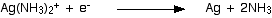Combining that with the half-equation for the oxidation of an aldehyde under alkaline conditions:. . . gives the overall equation: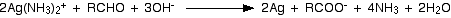```
```

Using Fehling's solution or Benedict's solution

Fehling's solution and Benedict's solution are variants of essentially the same thing. Both contain complexed copper(II) ions in an alkaline solution.

Fehling's solution contains copper(II) ions complexed with tartrate ions in sodium hydroxide solution. Complexing the copper(II) ions with tartrate ions prevents precipitation of copper(II) hydroxide.

Benedict's solution contains copper(II) ions complexed with citrate ions in sodium carbonate solution. Again, complexing the copper(II) ions prevents the formation of a precipitate - this time of copper(II) carbonate.

Both solutions are used in the same way. A few drops of the aldehyde or ketone are added to the reagent, and the mixture is warmed gently in a hot water bath for a few minutes.

 ketone No change in the blue solution. aldehyde The blue solution produces a dark red precipitate of copper(I) oxide.
```
```

Aldehydes reduce the complexed copper(II) ion to copper(I) oxide. Because the solution is alkaline, the aldehyde itself is oxidised to a salt of the corresponding carboxylic acid.

Note:  I have watched students do this reaction with aldehydes and Fehling's solution over many years. Getting the dark red precipitate described in all the books was actually pretty rare! A lot of imagination had to go in to spotting the red colour in amongst all the other colours you tend to get as well. It wasn't one of my favourite tests. I have never used Benedict's solution - perhaps that behaves better!

Methanal is such a powerful reducing agent that the copper(II) ions may be reduced to metallic copper - often seen as a very nice copper mirror on the tube.

The equations for these reactions are always simplified to avoid having to write in the formulae for the tartrate or citrate ions in the copper complexes. The electron-half-equations for both Fehling's solution and Benedict's solution can be written as:Combining that with the half-equation for the oxidation of an aldehyde under alkaline conditions:. . . gives the overall equation: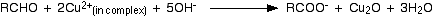Note:  If you are really alert, you might wonder where the hydroxide ions come from if you are talking about Benedict's solution, which is made alkaline using sodium carbonate. Sodium carbonate solution is alkaline because the carbonate ions react reversibly with water to produce hydroxide ions (and hydrogencarbonate ions).

```
```
 Questions to test your understanding If this is the first set of questions you have done, please read the introductory page before you start. You will need to use the BACK BUTTON on your browser to come back here afterwards. questions on the oxidation of aldehydes and ketones answers
```
```

Where would you like to go now?

To the aldehydes and ketones menu . . .

To the menu of other organic compounds . . .

To Main Menu . . .

```
```

© Jim Clark 2004 (modified November 2015)